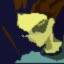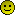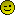###Author Topic: Fractal Effect[BB2D]  (Read 1874 times)

0 Members and 1 Guest are viewing this topic.

####mike_g

• Amiga 1200
••• Posts: 435
• Karma: 34##### Fractal Effect[BB2D]
« on: August 06, 2007 »
An animated fractal effect, I kind of made by accident.
Code: [Select]
`AppTitle "Fractals"Graphics 640, 480, 32, 2SetBuffer BackBuffer()Const recursions = 8Const base_length = 100Const base_lines = 3Const ox = 320Const oy = 240Const spawn = 2Const shrink# = 1.6Type lines Field x1, y1 Field x2, y2 Field length Field angle#End TypeGlobal off;deg_off = 360/spawnWhile Not KeyHit(1)    Cls off=off+1 Fractal() Flip Delay(1) WendFunction Fractal() length = base_length num_lines = base_lines deg_off = (360/base_lines)+off For i = 1 To base_lines l.lines = New lines l\x1=ox: l\y1=oy l\angle# = i * deg_off l\length = base_length l\x2 = l\x1+Sin(l\angle)*l\length l\y2 = l\y1+Cos(l\angle)*l\length Next For r = 1 To recursions re=200: gr=100: bl=100 Color re-(r*16), gr-(r*8), bl-(r*8) For l.lines = Each lines Line l\x1, l\y1, l\x2, l\y2 Next count = 0 For l.lines = Each lines count = count + 1 If r < recursions For i = 1 To spawn l2.lines = New lines l2\x1 = l\x2: l2\y1 = l\y2 l2\length = l\length/shrink# l2\angle = l\angle - (((spawn+1)*deg_off)/2) l2\angle = l2\angle + (i * deg_off) l2\x2 = l2\x1+Sin(l2\angle)*l2\length l2\y2 = l2\y1+Cos(l2\angle)*l2\length Next EndIf Delete l.lines ;delete the old line If r = 1 And count >= base_lines Then Exit If r > 1 And count >= base_lines*(spawn ^ (r-1)) Then Exit Next NextEnd Function`It could really do with some optimasation in that is doesent create then destroy each line each time the function is called.

####va!n

• Pentium
••• Posts: 1418
• Karma: 109##### Re: Fractal Effect[BB2D]
« Reply #1 on: August 06, 2007 »
Wow! Looks very cool!- hp EliteBook 8540p, 4 GB RAM, Windows 8.1 x64
- Asus P5Q, Intel Q8200, 6 GB DDR2, Radeon 4870, Windows 8.1 x64
http://www.secretly.de
Challenge Trophies Won:####mike_g

• Amiga 1200
••• Posts: 435
• Karma: 34##### Re: Fractal Effect[BB2D]
« Reply #2 on: August 06, 2007 »
Cheers, I'll see if I can get it running faster with some more stuff going on.

####Rbz

• Founder Member
••• Posts: 2731
• Karma: 485##### Re: Fractal Effect[BB2D]
« Reply #3 on: August 06, 2007 »
Very nice, sometimes it looks like trees

K++

####Jim

• Founder Member
••• Posts: 5301
• Karma: 402##### Re: Fractal Effect[BB2D]
« Reply #4 on: August 06, 2007 »
How come my mistakes don't look like that?Jim

####va!n

• Pentium
••• Posts: 1418
• Karma: 109##### Re: Fractal Effect[BB2D]
« Reply #5 on: August 06, 2007 »
@mike_g:
i just played a bit around and come up with this color style. Just replace your "color()" line with one of the followings:

Code: [Select]
` Color re-(r*16), gr-(r/8), bl-(r*8)`
Code: [Select]
` Color re-(r*16) Xor bl, gr-(r/8), bl-(r*8)`
- hp EliteBook 8540p, 4 GB RAM, Windows 8.1 x64
- Asus P5Q, Intel Q8200, 6 GB DDR2, Radeon 4870, Windows 8.1 x64
http://www.secretly.de
Challenge Trophies Won:####mike_g

• Amiga 1200
••• Posts: 435
• Karma: 34##### Re: Fractal Effect[BB2D]
« Reply #6 on: August 06, 2007 »
Hey, thats cool, makes it look a bit more plantlike. Altering the constants changes the effect quite a bit, its best not to set 'recursions' much higher tho, but I guess you guys will know that